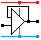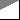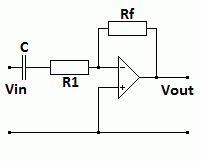Home

rOm
Quest
Glossary

Random
Page
Search
Site
Lush
Sim
Class
Subject
Images

Help
FAQ
Sign
Up
Log
In
 A Level     AC Circuits     >Active Filters<     Passive Filters     Tuned Circuits

# AC Circuits Active Filters

Site for Eduqas/WJEC - Go to the AQA site.

A Level    FiltersQuestions 0 to 38   -->  View All

## Inverting Op-Amp First Order Active Filters

• These filters add a capacitor to the usual inverting amplifier circuit.
• The capacitor is a frequency dependent component so the amplifier gain now depends on the frequency.

### Low Pass - Treble Cut• For DC and low frequencies, the capacitor has no effect.
• The DC and low frequency gain = -Rf / R1.
• Well above the breakpoint frequency, the capacitor
reactance is very low.
• At high frequencies, the gain drops to zero due
to the negative feedback through the capacitor.
• The input resistance equals R1.

### Treble Boost• At low frequencies, the capacitor has no effect.
• At low frequencies, the gain = -Rf / R1.
• At high frequencies the capacitor reduces R1.
• At high frequencies the gain increases.

### High Pass - Bass Cut• The capacitor blocks DC and low frequencies.
• The capacitor passes high frequencies easily.
• The amplifier provides gain.
• DC and low frequencies the gain = 0.
• Well above the breakpoint frequency, the gain = -Rf / R1.

### Bass Boost• For DC and low frequencies, the capacitor has no effect.
• Rf must be large.
• At low frequencies the gain is large = -Rf / R1.
• At high frequencies, Xc is low so the gain drops to zero.
• The input resistance equals R1

### Breakpoint Frequency Calculations

• These calculations apply to both passive and active filters.
• At the breakpoint frequency, the reactance of the capacitor equals the resistance of the resistor.

• R = Xc

• Xc = 1 / (2 π f C)

• R = 1 / (2 π f C)

• R f = 1 / (2 π C)

• f = 1 / (2 π R C)

• C = 1 / (2 π R f)

Subject Name     Level     Topic Name     Question Heading     First Name Last Name Class ID     User ID

 CloseEscapeX Q: qNum of last_q     Q ID: Question ID         Score: num correct/num attempts         Date Done

Question Text

image url

Help Text
Debug

• You can attempt a question as many times as you like.
• If you are logged in, your first attempt, each day, is logged.
• To improve your scores, come back on future days, log in and re-do the questions that caused you problems.
• In the grade book, you can delete your answers for a topic before re-doing the questions. Avoid deleting unless you intend re-doing the questions very soon.